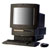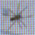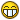# I just cant do this question!!! ARRRRRR!

3 posts / 0 newOffline
Last seen: 11 years 2 months ago
Joined: Dec 20 2003 - 10:38
Posts: 634
I just cant do this question!!! ARRRRRR!

This is really annoying me. I can't do the question below, or any like it. I can do collisions, relitive vectors, questions that are supposed to be harder...but when it comes to acceleration and velocity vectors I get stuck!

Question:

A particle has an intial veloctiy of i + 2j and is accelerating in the direction i + j. If the magnitude of the acceleration is 5(2^0.5), find the velocity vector and the speed of the particle after 2 seconds.

If anyone can explain how I should go about this kind of question I would be very grateful. I need the A in Jan!

Thanks

TOffline
Last seen: 16 years 1 month ago
Joined: Dec 20 2003 - 10:38
Posts: 71
An explantion?

This problem has two steps, calculating the actual acceleration vector from the direction and magnitude information and then calculating the velocity as it changes with accumulating acceleration.

To start, lets calculate the actual acceleration vector. We know the direction of acceleration is i+j, but don't know exact acceleration vector. But we do know the total magnitude. So we need to find an acceleration vector with direction i+j and magnitude 5(2^0.5). This vector will be of the form xi + xj which is acceleration in the direction of i+j, scaled by some unknown acceleration constant, x.

We know that the magnitude of the acceleration (the square root of the sum of the squares of the two components of acceleration is 5(2^0.5) so then,

(x^2 + x^2)^0.5 = 5(2^0.5).
(2 x^2)^0.5 = 5(2^0.5)
x(2^0.5) = 5(2^0.5)
so x = 5

Thus the acceleration vector is 5i + 5j

Now lets calculate the total velocity after some period of acceleration. The velocity is the initial velocity plus acceleration times time so that is v0 + at

In this case initial velocity is i + 2j so the velocity after two seconds is:
(i+2j) + 2*(5i+5j)
= (i+2j) + 10i+10j
= 11i + 12j

Does that make sense?Offline
Last seen: 11 years 2 months ago
Joined: Dec 20 2003 - 10:38
Posts: 634
ThanksThat made perfect sense and was the correct answer. I was doing it completly wrong...but I should be able to do those kind of questions now.